# Introduction

I took this tutorial from a reply I posted on this forum. Basically, the idea is we have some theoretical part, and we want to be able to detect if the part can be seen by a player. This has to be done in a local script because otherwise we can’t access the local player’s camera.

WARNING: This tutorial gets a little bit mathematically complex, but I try to explain it as thoroughly as possible.

# Finding the Part's Corners

First step is to be able to essentially create a polygon out of the part by projecting it’s vertices onto the camera. Before that though, we need to actually find the vertices:

``````-- Variables
local part = workspace.THEPart
local camera = workspace.CurrentCamera

-- Delay

-- Finding Vertices of Part
local vertices = {}
for i1, x in pairs({-0.5, 0.5}) do
for i2, y in pairs({-0.5, 0.5}) do
for i3, z in pairs({-0.5, 0.5}) do
local vertex = (part.CFrame * CFrame.new(x * part.Size.X, y * part.Size.Y, z * part.Size.Z)).Position
table.insert(vertices, vertex)
end
end
end

-- Displaying Vertices
local function displayVertices(vertices)
for i, vertex in pairs(vertices) do
local display = Instance.new("Part")
display.CFrame = CFrame.new(vertex)
display.Anchored = true
display.Size = Vector3.new(1, 1, 1)
display.Color = Color3.new(1, 0, 0)
display.Material = Enum.Material.Neon
display.Parent = workspace
end
end
displayVertices(vertices)
``````

And that should work nicely even if the part is rotated, since we did it with CFrames (or alternatively you could use the .LookVector, .RightVector, and .UpVector properties of the part).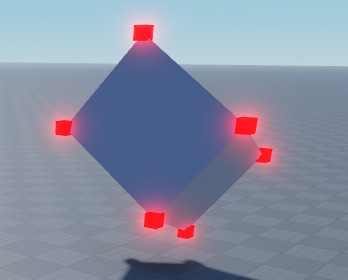# Converting Part Corners to a 2D Polygon

Now that we have our vertices, let’s create a polygon out of them. In order to do that, we need to first project each vertex to the camera, using camera:WorldToScreenPoint().

``````-- Displaying Camera Points
local screenGui = Instance.new("ScreenGui", playerGui); screenGui.Name = "DisplayCameraPointsGui"
local function displayCameraPoints(points)

-- Displaying Points
for i, point in pairs(points) do
local frame = Instance.new("Frame")
frame.Size = UDim2.fromOffset(10, 10)
frame.AnchorPoint = Vector2.new(0.5, 0.5)
frame.BackgroundColor3 = Color3.new(1, 0, 0)
frame.Position = UDim2.fromOffset(point.X, point.Y)
frame.Parent = screenGui
end

end

-- Turning Vertex List Into Polygon
local function createPolygon(vertices)

-- Finding Camera Points
local cameraPoints = {}
for i, vertex in pairs(vertices) do
local point, onScreen = camera:WorldToScreenPoint(vertex)
if point.Z >= 0 then -- don't include points that are behind the camera
table.insert(cameraPoints, Vector2.new(point.X, point.Y)) -- remove the z
end
end

-- Displaying Camera Points
displayCameraPoints(cameraPoints)

end

createPolygon(vertices)
``````

And thus we get something: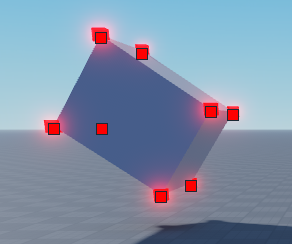Obviously we’ll have to do this on a loop but first we’ll just create a polygon with which we can reference the individual edges of. In order to do this, we will find the “convex hull” of our list of points.

Since this is something I’ve admittedly never done before and I just barely found out about this by looking it up, I will be following the method described in this video: Graham Scan Tutorial: Convex Hull of a Set of 2D Points - YouTube

The first step as mentioned in the video is to find the lowest point and order them based on the angle from that lowest point. In order to visualize the order of the points, I changed the displayCameraPoints() function to color them based on the order:

``````-- Displaying Camera Points
local screenGui = Instance.new("ScreenGui", playerGui); screenGui.Name = "DisplayCameraPointsGui"
local function displayCameraPoints(points)

-- Displaying Points
for i, point in pairs(points) do
local frame = Instance.new("Frame")
frame.Size = UDim2.fromOffset(10, 10)
frame.AnchorPoint = Vector2.new(0.5, 0.5)
frame.BackgroundColor3 = Color3.fromHSV(i / (#points * 4), 1, 1) -- to visualize the order of the points
frame.Position = UDim2.fromOffset(point.X, point.Y)
frame.Parent = screenGui
end

end
``````

And then in the createPolygon() function I orded the points as instructed in the video I linked and then moved the displayCameraPoints() function call underneath the ordering:

``````-- Turning Vertex List Into Polygon
local function createPolygon(vertices)

-- Finding Camera Points
local cameraPoints = {}
for i, vertex in pairs(vertices) do
local point, onScreen = camera:WorldToScreenPoint(vertex)
if point.Z >= 0 then -- don't include points that are behind the camera
table.insert(cameraPoints, Vector2.new(point.X, point.Y)) -- remove the z
end
end

-- Ordering Points
local startingPoint = cameraPoints
for i, point in pairs(cameraPoints) do
if point.Y > startingPoint.Y or (point.Y == startingPoint.Y and point.X > startingPoint.X) then -- remember the position goes down as y goes up
startingPoint = point
end
end

table.sort(cameraPoints, function(a, b)
if a == startingPoint then
return true
elseif b == startingPoint then
return false
end

-- Finding Rise and Run of A and B
local runA, runB = a.X - startingPoint.X, b.X - startingPoint.X
local riseA, riseB = a.Y - startingPoint.Y, b.Y - startingPoint.Y

-- Finding Negative Reciprocals
local recipA = if riseA ~= 0 then -runA / riseA else -math.huge
local recipB = if riseB ~= 0 then -runB / riseB else -math.huge

return recipA > recipB

end)

-- Displaying Camera Points
displayCameraPoints(cameraPoints)
print(cameraPoints)

end

createPolygon(vertices)
``````

And since this is what I get: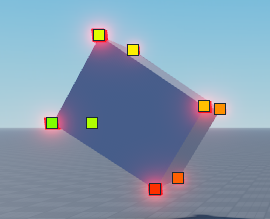I think it worked, so let’s move on. Next step is to loop through these ordered points and essentially get rid of all of the inside points. First we’ll create two tables, “convexHullPoints”, which will store the points for the new polygon, and “recentLeftTurns”, which will store the indices of each point that marks a turn left, so that way we can keep track of them in order to undo them if the next turn goes right.

After that, we loop through all the points, check if each turn is left or right using the determinant (calculation which I just found online) and if the turn is left, we add the index of the third point in the turn and move on. If the turn is right, we remove the amount of points from convexHullPoints equal to the amount of left turns, and then re-add the new point as it would’ve been removed. If the determinant is zero, we remove the point just before this one, since it would be creating a straight line anyway, but I don’t think the determinant would really ever be zero. Anywhere here’s the code for it:

``````-- Turning Vertex List Into Polygon
local function createPolygon(vertices)

-- Finding Camera Points
local cameraPoints = {}
for i, vertex in pairs(vertices) do
local point, onScreen = camera:WorldToScreenPoint(vertex)
if point.Z >= 0 then -- don't include points that are behind the camera
table.insert(cameraPoints, Vector2.new(point.X, point.Y)) -- remove the z
end
end

-- Ordering Points
local startingPoint = cameraPoints
for i, point in pairs(cameraPoints) do
if point.Y > startingPoint.Y or (point.Y == startingPoint.Y and point.X > startingPoint.X) then -- remember the position goes down as y goes up
startingPoint = point
end
end

table.sort(cameraPoints, function(a, b)
if a == startingPoint then
return true
elseif b == startingPoint then
return false
end

-- Finding Rise and Run of A and B
local runA, runB = a.X - startingPoint.X, b.X - startingPoint.X
local riseA, riseB = a.Y - startingPoint.Y, b.Y - startingPoint.Y

-- Finding Negative Reciprocals
local recipA = if riseA ~= 0 then -runA / riseA else -math.huge
local recipB = if riseB ~= 0 then -runB / riseB else -math.huge

return recipA > recipB

end)

-- Creating Convex Hull Polygon
local convexHullPoints = {}
local recentLeftTurns = {}

for i, point in pairs(cameraPoints) do

table.insert(convexHullPoints, point)

-- Checking Last Two Points
if #convexHullPoints >= 3 then

-- Finding Points
local point1 = convexHullPoints[#convexHullPoints - 2]
local point2 = convexHullPoints[#convexHullPoints - 1]
local point3 = point

-- Checking Direction of Turn
local det = (point1.X*point2.Y + point2.X*point3.Y + point3.X*point1.Y) - (point2.Y*point3.X + point3.Y*point1.X + point1.Y*point2.X) -- determinant shortcut
if det < 0 then
print('left turn')
table.insert(recentLeftTurns, #convexHullPoints)
elseif det > 0 then
print('right turn')

-- Removing All Consecutive Left Turns Before
print(recentLeftTurns)
for i = 1, math.max(#recentLeftTurns, 2) do
table.remove(convexHullPoints)
end
table.insert(convexHullPoints, point) -- re-adding new point
recentLeftTurns = {}

elseif det == 0 then
table.remove(convexHullPoints, (#convexHullPoints - 1))
end

end

end

-- Returning Polygon
return convexHullPoints

end

local polygon = createPolygon(vertices)
displayCameraPoints(polygon)
``````

And now you can see we’ve removed the inner points and essentially have our polygon.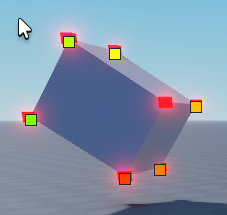Now we just need to detect the actual collision between this polygon and the screen itself . . .
But before that let’s define a similar list of vertices for the camera as a polygon. This will just consist of all of the corners of the screen, from the bottom right around counter-clockwise.

``````-- Creating Polygons
local partPolygon = createPolygon(vertices)
local camPolygon = {
Vector2.new(screenGui.AbsoluteSize.X, screenGui.AbsoluteSize.Y),
Vector2.new(screenGui.AbsoluteSize.X, 0),
Vector2.new(0, 0),
Vector2.new(0, screenGui.AbsoluteSize.Y)
}
``````

And if you run the same displayCameraPoints() function on the camPolygon table, then you can see the points line around the corners of the screen, not including the gui inset.

# Detecting if the Polygon Collides With the Camera Box

And now we’re ready for the actual detection part. You can also put this in a loop now. Here’s a few changes I made:

I added a remove time to the displayCameraPoints() function, so we can display the points in a loop without keeping the old points:

``````-- Displaying Camera Points
local screenGui = Instance.new("ScreenGui", playerGui); screenGui.Name = "DisplayCameraPointsGui"
local function displayCameraPoints(points, timeDelay)

-- Displaying Points
for i, point in pairs(points) do
local frame = Instance.new("Frame")
frame.Size = UDim2.fromOffset(10, 10)
frame.AnchorPoint = Vector2.new(0.5, 0.5)
frame.BackgroundColor3 = Color3.fromHSV(i / (#points * 4), 1, 1) -- to visualize the order of the points
frame.Position = UDim2.fromOffset(point.X, point.Y)
frame.Parent = screenGui

if timeDelay then
frame:Destroy()
end)
end
end

end
``````

And then I created a main loop that we’ll use to organize the actual functionality (alternatively you could use a RunService loop):

``````-- Main Loop
while true do

-- Creating Polygons
local partPolygon = createPolygon(vertices)
local camPolygon = {
Vector2.new(screenGui.AbsoluteSize.X, screenGui.AbsoluteSize.Y),
Vector2.new(screenGui.AbsoluteSize.X, 0),
Vector2.new(0, 0),
Vector2.new(0, screenGui.AbsoluteSize.Y)
}
displayCameraPoints(partPolygon, 0)

end
``````

I decided to play around and see what it’d look like if the screen were black and I could see the points on top, so here’s that: https://gyazo.com/cf0024c70ae1b407a798cd40ccce29cf

But so far, little to no lag.

The next step is detecting whether or not the polygons are intersecting. I will be following this article: Collision Detection Using the Separating Axis Theorem

Surprisingly this step was a bit easier after I got it figured out. I created a function to detect the intersection between the two polygons. If either polygon has 2 points or less (in the case that the part is behind the camera, or partially so), then obviously we can’t really do much with that so we just return false. I then looped through every point in poly1, keeping track of the last point before it, and found the normal, or the outward direction perpendicular to the edge.

I then went through all of the points of both polygons and projected them to this normal line using their dot product. It’s sort of like creating a shadow onto the axis that is the normal direction. Afterwards, I found the minimum and maximum dot products for both polygons and checked if the “shadows” overlapped. If they didn’t I immediately returned false, and if every one of the shadows overlapped one another on every normal axis, I returned true at the end. On top of this, I ran the function both ways, first with the part being poly1 and the camera being poly2, and then again with them swapped, so as to check the normals of both polygons.

``````-- Detecting Intersection
local function detectIntersection(poly1, poly2)
if #poly1 < 3 or #poly2 < 3 then return false end

-- Looping Normals of First Polygon
local lastPoint = poly1[#poly1]
for i, point in pairs(poly1) do

-- Variables
local edgeDir = (point - lastPoint).Unit
local normal = Vector2.new(edgeDir.Y, -edgeDir.X)

-- Projecting Polygon Points
local projected1 = {}
local projected2 = {}

for i2, point in pairs(poly1) do
local projectedPoint = point:Dot(normal) -- this is like casting a shadow onto the axis that the "normal" creates
table.insert(projected1, projectedPoint)
end

for i2, point in pairs(poly2) do
local projectedPoint = point:Dot(normal)
table.insert(projected2, projectedPoint)
end

-- Finding Minimums and Maximums
local min1, max1 = projected1, projected1
local min2, max2 = projected2, projected2
for i2, dot in pairs(projected1) do
min1, max1 = math.min(dot, min1), math.max(dot, max1)
end
for i2, dot in pairs(projected2) do
min2, max2 = math.min(dot, min2), math.max(dot, max2)
end

-- Checking Overlap
if max1 < min2 or max2 < min1 then
return false -- if any of the normal projections don't overlap then the polygons don't either
end

-- Setting Last Point
lastPoint = point

end

-- Returning
return true -- only returns if all normal projections go through as true

end

-- Main Loop
while true do

-- Creating Polygons
local partPolygon = createPolygon(vertices)
local camPolygon = {
Vector2.new(screenGui.AbsoluteSize.X, screenGui.AbsoluteSize.Y),
Vector2.new(screenGui.AbsoluteSize.X, 0),
Vector2.new(0, 0),
Vector2.new(0, screenGui.AbsoluteSize.Y)
}
--displayCameraPoints(partPolygon, 0)

-- Detecting Intersection
local doesIntersect1 = detectIntersection(partPolygon, camPolygon)
local doesIntersect2 = detectIntersection(camPolygon, partPolygon)

local doesIntersect = doesIntersect1 and doesIntersect2
print(doesIntersect)

end
``````

Here’s a demonstration: https://gyazo.com/58cb135dd901fb11fcc4b13cb281a81f

Here’s the final, ultimately beefy, script:

``````-- Player Variables
local player = game.Players.LocalPlayer
local playerGui = player.PlayerGui

-- Variables
local part = workspace.THEPart
local camera = workspace.CurrentCamera

-- Delay

-- Finding Vertices of Part
local vertices = {}
for i1, x in pairs({-0.5, 0.5}) do
for i2, y in pairs({-0.5, 0.5}) do
for i3, z in pairs({-0.5, 0.5}) do
local vertex = (part.CFrame * CFrame.new(x * part.Size.X, y * part.Size.Y, z * part.Size.Z)).Position
table.insert(vertices, vertex)
end
end
end

-- Displaying Vertices
local function displayVertices(vertices)
for i, vertex in pairs(vertices) do
local display = Instance.new("Part")
display.CFrame = CFrame.new(vertex)
display.Anchored = true
display.Size = Vector3.new(1, 1, 1)
display.Color = Color3.new(1, 0, 0)
display.Material = Enum.Material.Neon
display.Parent = workspace
end
end
--displayVertices(vertices)

-- Displaying Camera Points
local screenGui = Instance.new("ScreenGui", playerGui); screenGui.Name = "DisplayCameraPointsGui"
local function displayCameraPoints(points, timeDelay)

-- Displaying Points
for i, point in pairs(points) do
local frame = Instance.new("Frame")
frame.Size = UDim2.fromOffset(10, 10)
frame.AnchorPoint = Vector2.new(0.5, 0.5)
frame.BackgroundColor3 = Color3.fromHSV(i / (#points * 4), 1, 1) -- to visualize the order of the points
frame.Position = UDim2.fromOffset(point.X, point.Y)
frame.Parent = screenGui

if timeDelay then
frame:Destroy()
end)
end
end

end

-- Turning Vertex List Into Polygon
local function createPolygon(vertices)

-- Finding Camera Points
local cameraPoints = {}
for i, vertex in pairs(vertices) do
local point, onScreen = camera:WorldToScreenPoint(vertex)
if point.Z >= 0 then -- don't include points that are behind the camera
table.insert(cameraPoints, Vector2.new(point.X, point.Y)) -- remove the z
end
end

-- Ordering Points
local startingPoint = cameraPoints
for i, point in pairs(cameraPoints) do
if point.Y > startingPoint.Y or (point.Y == startingPoint.Y and point.X > startingPoint.X) then -- remember the position goes down as y goes up
startingPoint = point
end
end

table.sort(cameraPoints, function(a, b)
if a == startingPoint then
return true
elseif b == startingPoint then
return false
end

-- Finding Rise and Run of A and B
local runA, runB = a.X - startingPoint.X, b.X - startingPoint.X
local riseA, riseB = a.Y - startingPoint.Y, b.Y - startingPoint.Y

-- Finding Negative Reciprocals
local recipA = if riseA ~= 0 then -runA / riseA else -math.huge
local recipB = if riseB ~= 0 then -runB / riseB else -math.huge

return recipA > recipB

end)

-- Creating Convex Hull Polygon
local convexHullPoints = {}
local recentLeftTurns = {}

for i, point in pairs(cameraPoints) do

table.insert(convexHullPoints, point)

-- Checking Last Two Points
if #convexHullPoints >= 3 then

-- Finding Points
local point1 = convexHullPoints[#convexHullPoints - 2]
local point2 = convexHullPoints[#convexHullPoints - 1]
local point3 = point

-- Checking Direction of Turn
local det = (point1.X*point2.Y + point2.X*point3.Y + point3.X*point1.Y) - (point2.Y*point3.X + point3.Y*point1.X + point1.Y*point2.X) -- determinant shortcut
if det < 0 then
table.insert(recentLeftTurns, #convexHullPoints)
elseif det > 0 then

-- Removing All Consecutive Left Turns Before
for i = 1, math.max(#recentLeftTurns, 2) do
table.remove(convexHullPoints)
end
table.insert(convexHullPoints, point) -- re-adding new point
recentLeftTurns = {}

elseif det == 0 then
table.remove(convexHullPoints, (#convexHullPoints - 1))
end

end

end

-- Returning Polygon
return convexHullPoints

end

-- Detecting Intersection
local function detectIntersection(poly1, poly2)
if #poly1 < 3 or #poly2 < 3 then return false end

-- Looping Normals of First Polygon
local lastPoint = poly1[#poly1]
for i, point in pairs(poly1) do

-- Variables
local edgeDir = (point - lastPoint).Unit
local normal = Vector2.new(edgeDir.Y, -edgeDir.X)

-- Projecting Polygon Points
local projected1 = {}
local projected2 = {}

for i2, point in pairs(poly1) do
local projectedPoint = point:Dot(normal) -- this is like casting a shadow onto the axis that the "normal" creates
table.insert(projected1, projectedPoint)
end

for i2, point in pairs(poly2) do
local projectedPoint = point:Dot(normal)
table.insert(projected2, projectedPoint)
end

-- Finding Minimums and Maximums
local min1, max1 = projected1, projected1
local min2, max2 = projected2, projected2
for i2, dot in pairs(projected1) do
min1, max1 = math.min(dot, min1), math.max(dot, max1)
end
for i2, dot in pairs(projected2) do
min2, max2 = math.min(dot, min2), math.max(dot, max2)
end

-- Checking Overlap
if max1 < min2 or max2 < min1 then
return false -- if any of the normal projections don't overlap then the polygons don't either
end

-- Setting Last Point
lastPoint = point

end

-- Returning
return true -- only returns if all normal projections go through as true

end

-- Main Loop
while true do

-- Creating Polygons
local partPolygon = createPolygon(vertices)
local camPolygon = {
Vector2.new(screenGui.AbsoluteSize.X, screenGui.AbsoluteSize.Y),
Vector2.new(screenGui.AbsoluteSize.X, 0),
Vector2.new(0, 0),
Vector2.new(0, screenGui.AbsoluteSize.Y)
}
--displayCameraPoints(partPolygon, 0)

-- Detecting Intersection
local doesIntersect1 = detectIntersection(partPolygon, camPolygon)
local doesIntersect2 = detectIntersection(camPolygon, partPolygon)

local doesIntersect = doesIntersect1 and doesIntersect2
print(doesIntersect)

end
``````

And here’s the place file:
part on screen test.rbxl (44.2 KB)

# In Summary

I’m not sure how this method would work in a RunService or other rapid loop, especially with multiple parts in consideration, but it should work for 100% of situations in which at least part of a part is on the screen. If you want to see if an entire part is on the screen, you could just run camera:WorldToScreenPoint() for each vertex of the part and return false if any of them aren’t visible to the player.

Also a small note, in this attempt I used :WorldToScreenPoint() instead of :WorldToViewportPoint(). There was no real reason for this, I just unintentionally swapped one in place for the other. As far as I can tell the main difference is whether or not it ignores the gui inset on the top of the screen.

24 Likes

Thanks for the tutorial! The tutorial itself is great, I’ll be using this in one of my upcoming games1 Question tho kinda important for my game, is there a way to only print if the part is ON the screen with no parts blocking the view of the part? for example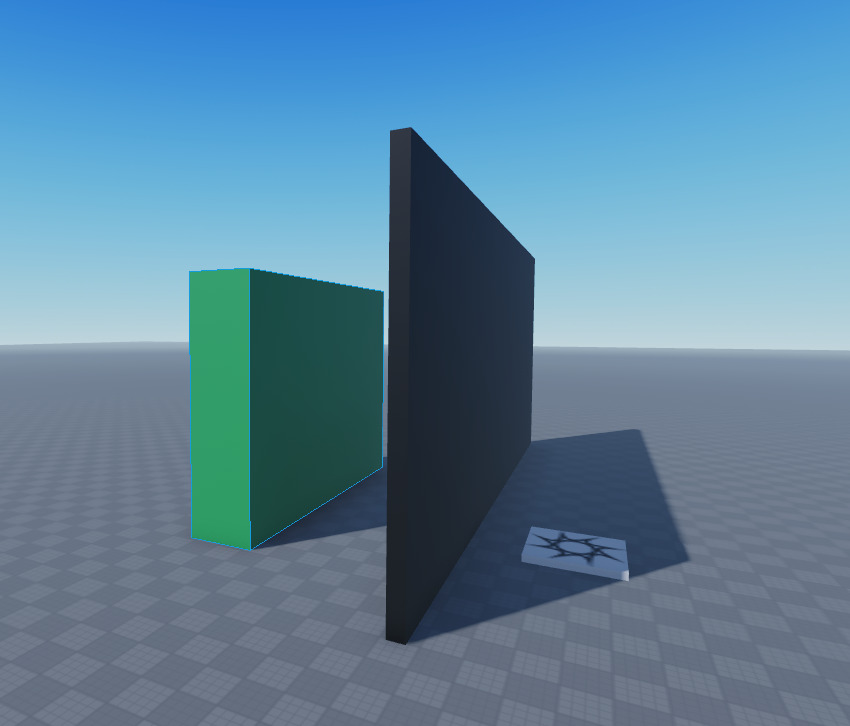I want the black wall to block it from being able to see it, but in this demo it just says the green part is on screen even with the black wall being in front.

If you can help me achieve this thank you so much

1 Like

Ooh that’s a good point. I didn’t consider that. I’m not sure if there’s a lag-efficient way of checking for parts in front of the part, but as a primitive and somewhat half-solution, you could simply raycast from the camera’s position to the center of the part and check if the raycast result.Instance is equal to the part. There’s a lot of situations that might not account for, but it should provide at least a lag-efficient solution that works in a few cases. Alternatively you could raycast to the vertices of the part for a wider check, but you might need to raycast inward towards the part slightly from each corner just to make sure the rays actually hit.

As for an absolute solution that’s right 100% of the time, I’m sure it exists and probably involves some sort of layering multiple polygons for every part, which obviously isn’t going to work, both for our sanity and for our computer’s processing power.